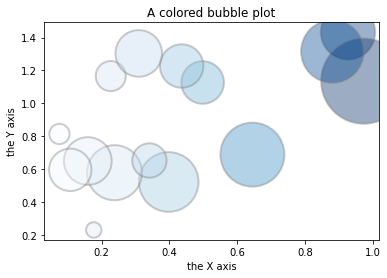A bubble plot is a scatterplot with a third dimension: the size of the markers. It is even possible to add a fourth dimension using colors. It can be done using the `cmap` argument of the `scatter()` function of matplotlib.

``````# libraries
import matplotlib.pyplot as plt
import numpy as np
import seaborn as sns

# create data
x = np.random.rand(15)
y = x+np.random.rand(15)
z = x+np.random.rand(15)
z=z*z

# Change color with c and transparency with alpha.
# I map the color to the X axis value.
plt.scatter(x, y, s=z*2000, c=x, cmap="Blues", alpha=0.4, edgecolors="grey", linewidth=2)

# Add titles (main and on axis)
plt.xlabel("the X axis")
plt.ylabel("the Y axis")
plt.title("A colored bubble plot")

# Show the graph
plt.show()``````## Contact & Edit

👋 This document is a work by Yan Holtz. You can contribute on github, send me a feedback on twitter or subscribe to the newsletter to know when new examples are published! 🔥